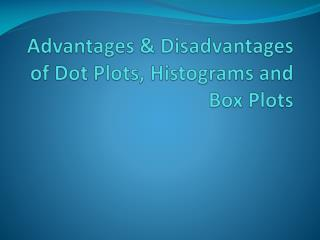# Advantages & Disadvantages of Dot Plots, Histograms and Box Plots - PowerPoint PPT PresentationDownload PresentationDownload Presentation- - - - - - - - - - - - - - - - - - - - - - - - - - - E N D - - - - - - - - - - - - - - - - - - - - - - - - - - -
##### Presentation Transcript

1. Warm-Up Joshua, a sophomore at Hoover High School, usually goes to bed around 11:00 p.m. and gets up around 8:00 a.m. to get ready for school. That means that he gets about 9 hours of sleep on a school night. He decided to investigate the statistical question: How many hours per night does a sophomore usually sleep when they have school the next day? Joshua surveyed 20 sophomores.  The following data set represents the average number of hours each student sleeps on a school night: {𝟕 𝟖 𝟓 𝟗 𝟗 𝟗 𝟕 𝟕 𝟏𝟎 𝟏𝟎 𝟏𝟏 𝟗 𝟖 𝟖 𝟖 𝟏𝟐 𝟔 𝟏𝟏 9𝟏𝟎} Make a dot plot, histogram and box plot to display the data.

2. Check-point! • Who would you have surveyed to answer the statistical question? • Which graph best illustrates the data?

3. The Statistical Process… • is a problem-solving process consisting of four steps: 1. formulating a statistical question that anticipates variability and can be answered by data 2. designing and implementing a plan that collects appropriate data. 3. analyzing the data by graphical and/or numerical methods. 4. interpreting the analysis in the context of the original question

4. Variables • Read the following statistical questions and determine whether the question is categorical or numerical. a. Do professors of math get paid more than professors of science? b. Do women live longer than men? c. What is the language most commonly spoken at home amongst people in South Florida? d. What is the length of students’ feet in Ms. Moe’s class? e. What is the favorite sport of students atMajorly High School? f. What is the post code of students that attend Flamingo Middle School?

5. Why is some data that contain numbers, such as the post codes considered categorical?

6. Types of Graphs: • Dot Plot • Histogram • Box Plot

7. Dot Plot • A dot plot is a graphic display using dots and a simple scale to compare the frequency within categories or groups.

8. Dot Plot(continued) • A dot plot is useful for relatively small sets of data. • Dot plots clearly display clusters/gaps of data and outliers. • In dot plots the frequency axis is not necessary but you need to count to find the frequency in each stack of dots, and they can be hard to construct and interpret for data sets with many points. • Can be used with numerical and categorical data.

9. Histogram • A histogram is a type of graph that shows the frequency distribution of data within equal intervals (thus, there are no spaces between the bars).

10. Histogram(continued) • It shows the number of values within an interval and not the actual values. • You can graph huge data sets easily with histograms. • Used only for numerical data. • You could change the intervals of the histogram to see which gives a better description of the data.

11. Box-Plots • The box plot is a standardized way of displaying the distribution of data based on the minimum, first quartile, median, third quartile, and maximum of the data set.

12. Box-Plots(continued) • A box plot is a good way to summarize large amounts of data. • It displays the range and distribution of data along a number line. • Box plots provide some indication of the data’s symmetry and skew-ness. • Box plots show outliers. • Original data is not clearly shown in the Box Plot; also, mean and mode cannot be identified in a Box Plot. • Can be used only with numerical data.

13. Remember: • Graphs must always be clearly labeled. • Changing the scales in a graph can make the data look very different; ultimately changing the impression that the graph makes. • When comparing two or more sets of data, the scales must be consistent; otherwise, it is difficult to compare the data.

14. What Graph Would You Choose? The following lists different data sets, which graphical representation would best illustrate the data? Explain. • Comparison of the annual snow fall between two snowboarding resorts over several years. • The amount of time spent watching TV, in hours, of 200 participants. • Wind speed of a wind-mill farm in a three-week period. • Students’ favorite summer-time activity.